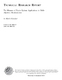## The Measure of Pareto Optima: Applications to Multi-objective MetaheuristicsThis article describes a set function that maps a series of Paretooptimal points to a scalar quantity. The Pareto optima constitutea maximal set of points in $k$-dimensional space. A proof ispresented that shows that the maximization of this scalar valueconstitutes the necessary and sufficient condition for itsarguments to be Pareto optimal solutions of a discrete,multi-objective, optimization problem. This scalar quantity isbased on a Lebesgue measure and can be used as the objectivefunction in various metaheuristics such as simulated annealing(SA) to induce convergence in probability to the Pareto optima. Anefficient algorithm that calculates this Lebesgue measure is alsopresented with an analysis of its complexity. Issues regarding useof the theoretical result as a baseline for developing otheruseful heuristics as well as applications and other considerationsare discussed.<P><center><I>This report is Copyright 2003 by Springer-Verlag.</I></center>